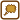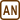## Gravitational and electric force acting on particles

Calculate the force by which two α-particles repel each other when their distance is 10−13 m and compare this force with the gravitational force. The charge of an α-particle is 3.2·10−19 C and its mass is 6.68·10−27 kg.

• #### Hint: gravitational and electric force

This relation applies for the value of the electric force:

$F_e\,=\,\frac{1}{4 \pi \varepsilon_0}\,\frac{Q_1 Q_2}{r^2}\,,$

where Q1, Q2 are the values of charges and r is the distance of the charges that act upon eachother.

This applies for the gravitational force:

$F_g\,=\,\kappa \,\frac{m_1 m_2}{r^2}\,,$

where m1, m2 are masses of charges that act upon eachother.

• #### Solution

We will calculate the value of electric force that the particles act on each other with using Coulomb law:

$F_e\,=\,\frac{1}{4 \pi \varepsilon_0}\,\frac{Q_1 Q_2}{r^2}\,.$

Since both particles are charged with the same charge Q, we can simplify the equation:

$F_e\,=\,\frac{1}{4 \pi \varepsilon_0}\,\frac{Q^2}{r^2}\,.$

We will calculate the value of the gravitational force using Newton’s law. Since both particles have the same mass m, we can simplify the equation once again:

$F_g\,=\,\kappa \,\frac{m_1 m_2}{r^2}\,=\,\kappa \,\frac{m^2}{r^2}\,.$

Next, we will find the ratio of both forces to see how many times is one force bigger than the other.

$\frac{F_e}{F_g}\,=\, \frac{\frac{1}{4 \pi \varepsilon_0}\,\frac{Q^2}{r^2}}{ \kappa\,\frac{m^2}{r^2}}$

$\frac{F_e}{F_g}\,=\, \frac{1}{4 \pi \varepsilon_0\kappa}\,\frac{Q^2r^2}{m^2r^2}\,=\, \frac{1}{4 \pi \varepsilon_0\kappa}\,\frac{Q^2}{m^2}$

We can see that the ratio of the electric and the gravitational force is independent of particle distance.

• #### List of known information and numerical calculation

r = 10−13 m

Q = 3.2·10−19 C

m = 6.68·10−27 kg

Fe = ? (N)

Fg = ? (N)

From tables:

ε0 = 8.85·10−12 C2N−1m−2

κ = 6.67·10−11 m3kg−1s−2

$\begin{eqnarray} F_e\,&=&\,\frac{1}{4 \pi \varepsilon_0}\,\frac{Q^2}{r^2}\,=\,\frac{1}{4 \pi \cdot 8.85{\cdot}10^{-12}}\cdot\frac{\left(3.2 {\cdot} 10^{-19}\right)^2}{\left(10^{-13}\right)^2}\,=\,9.2{\cdot}10^{-2}\,\mathrm{N}.\\ F_g\,&=&\,\kappa \,\frac{m^2}{r^2}\,=\,6.67{\cdot}10^{-11} \cdot\frac{\left(6.68 {\cdot} 10^{-27}\right)^2}{\left(10^{-13}\right)^2}\,=\, 3.0 {\cdot} 10^{-37}\,\mathrm{N}.\\ \frac{F_e}{F_g} \,&=&\, \frac{1}{4 \pi \varepsilon_0\kappa}\,\frac{Q^2}{m^2}\,=\,\frac{1}{4 \pi \cdot 8.85{\cdot}10^{-12}\cdot6.67{\cdot}10^{-11}}\cdot\frac{\left(3.2 {\cdot} 10^{-19}\right)^2}{\left(6.68{\cdot}10^{-27}\right)^2}\\ \frac{F_e}{F_g}\,&=&\,3{\cdot}10^{35} \end{eqnarray}$

The electric force is much larger than the gravitational force in these conditions.

α-particles repel with force of

$F_e\,=\,\frac{1}{4 \pi \varepsilon_0}\,\frac{Q^2}{r^2}\,=\,9.2 {\cdot} 10^{-2}\,\mathrm{N}.$

The value of the gravitational force is

$F_g\,=\,\kappa \,\frac{m^2}{r^2}\,=\,3.0 {\cdot} 10^{-37}\,\mathrm{N}.$

The ratio of the electric and the gravitational force is

$\frac{F_e}{F_g} \,=\, \frac{1}{4 \pi \varepsilon_0\,\kappa}\,\frac{Q^2}{m^2}=\,3{\cdot} 10^{35}.$

The electric force is thus much larger than the gravitational force.×Original source: Kohout, J. (2010). Studijní materiály ke cvičením z Elektřiny a magnetismu. Interní materiál, Plzeň.